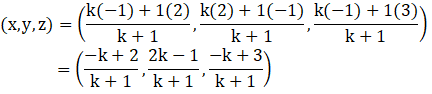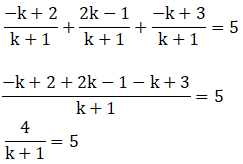Find the ratio in which the line segment joining the points (2, -1, 3) and (-1, 2, 1) is divided by the plane x + y + z = 5.

Asked by Sakshi | 1 year ago |  135

##### Solution :-

Given:

The points(2, -1, 3) and (-1, 2, 1)

Let C(x, y, z) be any point on the given plane and C divides AB in ratio k: 1

Then, m = k and n = 1

A(2, -1, 3) and B(-1, 2, 1)

Coordinates of C are:On comparing we get,

$$\dfrac{ [-k + 2] }{ [k + 1]}$$ = x

$$\dfrac{ [2k – 1] }{ [k + 1]}$$ = y

$$\dfrac{ [-k + 3]} { [k + 1]}$$ = z

We know that x + y + z = 55(k + 1) = 4

5k + 5 = 4

5k = 4 – 5

5k = – 1

k = $$\dfrac{-1}{5}$$

We can say that, the plane divides AB externally in the ratio 1:5

Answered by Aaryan | 1 year ago

### Related Questions

#### A(1, 2, 3), B(0, 4, 1), C(-1, -1, -3) are the vertices of a triangle ABC. Find the point in which the bisector of the

A(1, 2, 3), B(0, 4, 1), C(-1, -1, -3) are the vertices of a triangle ABC. Find the point in which the bisector of the angle ∠BAC meets BC.

#### The mid-points of the sides of a triangle ABC are given by (-2, 3, 5), (4, -1, 7) and (6, 5, 3). Find the coordinates

The mid-points of the sides of a triangle ABC are given by (-2, 3, 5), (4, -1, 7) and (6, 5, 3). Find the coordinates of A, B and C.

#### If the points A(3, 2, -4), B(9, 8, -10) and C(5, 4, -6) are collinear, find the ratio in which C divided AB.

If the points A(3, 2, -4), B(9, 8, -10) and C(5, 4, -6) are collinear, find the ratio in which C divided AB.